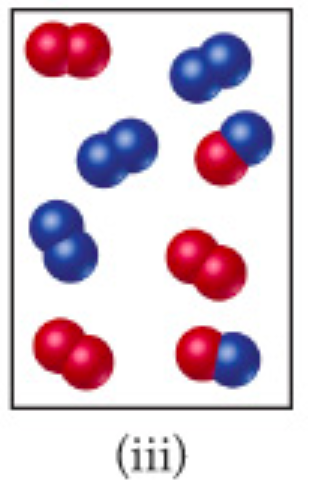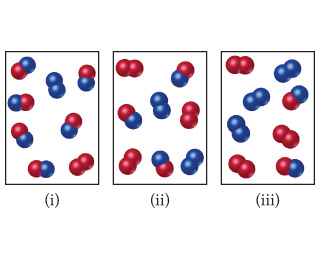# Problem: The reaction A2 + B2 ⇌ 2 AB has an equilibrium constant Kc =1.5. The following diagrams represent reaction mixtures containing A2 molecules (red), B2 molecules (blue), and AB molecules.For mixture (iii), how will the reaction proceed to reach equilibrium?

###### FREE Expert Solution

We are being asked to determine the condition for mixture (iii) to reach equilibrium.We will use the reaction quotient, Q, to determine if the chemical reaction would be at equilibrium or not.

The formula for Q is:

Depending on if Q is greater than or less than K, our reaction will shift to attain equilibrium by reaching the equilibrium constant K:

99% (171 ratings)###### Problem Details

The reaction A2 + B2 ⇌ 2 AB has an equilibrium constant Kc =1.5. The following diagrams represent reaction mixtures containing A2 molecules (red), B2 molecules (blue), and AB molecules.

For mixture (iii), how will the reaction proceed to reach equilibrium?